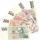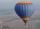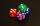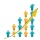# Recursion squares

In the square ABCD is inscribed a square so that its vertices lie at the centers of the sides of the square ABCD.The procedure of inscribing square is repeated this way. Side length of square ABCD is a = 22 cm.

Calculate:
a) the sum of perimeters of all squares
b) the sum of area of all squares

Result

Σ p =  300.45 cm
Σ S =  968 cm2

#### Solution:

$p_1 = 4\cdot 22 \ \\ q_p = \dfrac{ 1}{ \sqrt2} \ \\ \Sigma p = 4\cdot 22 \cdot \dfrac{ 1} { 1- \dfrac{ 1}{ \sqrt2}} = 300.45 \ \text { cm }$
$\ \\ S_1 = 22^2 \ \\ q_S = \dfrac{ 1}{ 2} \ \\ \Sigma S = 22^2 \cdot \dfrac{ 1} { 1- \dfrac{ 1}{ 2}} = 968 \ cm^2$

Leave us a comment of this math problem and its solution (i.e. if it is still somewhat unclear...):Be the first to comment!#### Following knowledge from mathematics are needed to solve this word math problem:

Pythagorean theorem is the base for the right triangle calculator. See also our trigonometric triangle calculator.

## Next similar math problems:

1. InfinityIn a square with side 18 is inscribed circle, in circle is inscribed next square, again circle and so on to infinity. Calculate the sum of area of all these squares.
2. Coordinates of square verticesI have coordinates of square vertices A / -3; 1/and B/1; 4 /. Find coordinates of vertices C and D, C 'and D'. Thanks Peter.
3. To the cinemaJane at 8 am got message that all 1093 school pupils will go to the cinema. Within 20 min she said it to the three friends. Each of them again for 20 minutes said to the other three. In this way the message spread further. At what time all the children in.
4. Miraculous treeMiraculous tree grows so fast that the first day increases its height by half the total height of the second day by the third, the third day by a quarter, etc. How many times will increase its height after 6 days?
5. Infinite decimalImagine the infinite decimal number 0.99999999 .. ... ... ... That is a decimal and her endless serie of nines. Determine how much this number is less than the number 1. Thank you in advance.
6. Before yesterdayHe merchant adds a sale sign in his shop window to the showed pair of shoes in the morning: "Today by p% cheaper than yesterday. " After a while, however, he decided that the sign saying: "Today 62.5% cheaper than the day before yesterday". Determine the n
7. Saving per centsThe first day I save 1 cent and every next day cent more. How many I saved per year (365 days)?
8. FractionFraction ? write as fraction a/b, a, b is integers numerator/denominator.
9. Sum of seriesDetermine the 6-th member and the sum of a geometric series: 5-4/1+16/5-64/25+256/125-1024/625+....
10. Hot air balloonHot air balloon ascends 25 meters up for a minute after launch. Every minute ascends 75 percent of the height which climbed in the previous minute. a) how many meters ascends six minutes after takeoff? b) what is the overall height 10 minutes after launch
11. Decimal to fractionWrite decimal number 8.638333333 as a fraction A/B in the basic form. Given decimal has infinite repeating figures.
12. Ten dicesWhen you hit ten dices at the same time you get average 35. How much do you hit if every time you get six, you're throwing the dice again?
13. Series and sequencesFind a fraction equivalent to the recurring decimal? 0.435643564356
14. Population growthHow many people will be on Earth from two people for 5,000 years, if every couple has always 4 children, (2 boys and 2 girls) at the age of 25-35, and every man will live 75 years?
15. Infinite sum of areasAbove the height of the equilateral triangle ABC is constructed an equilateral triangle A1, B1, C1, of the height of the equilateral triangle built A2, B2, C2, and so on. The procedure is repeated continuously. What is the total sum of the areas of all tri
16. Sides of right angled triangleOne leg is 1 m shorter than the hypotenuse, and the second leg is 2 m shorter than the hypotenuse. Find the lengths of all sides of the right-angled triangle.
17. A rhombusA rhombus has sides of length 10 cm, and the angle between two adjacent sides is 76 degrees. Find the length of the longer diagonal of the rhombus.